# GMAT Math : Median

## Example Questions

← Previous 1 3

### Example Question #1 : Calculating Median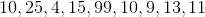What is the median of the data set?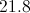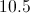Explanation:

Write the numbers in order from smallest to largest.  The median is the middle number, which is 11.

### Example Question #1 : Calculating Median

What is the median of the following numbers?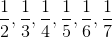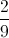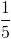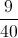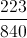Explanation:

The median of a data set with an even number of elements is the mean of its two middle elements, when ranked. The set is already ranked, so just find the mean of middle elementsand: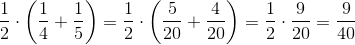### Example Question #1 : Calculating Median

Give the median of the following data set in terms of: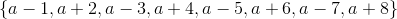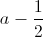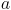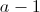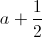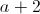Explanation:

The data set can be arranged from least to greatest as follows: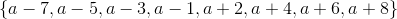The median of a data set with eight elements is the mean of its fourth-highest and fourth-lowest elements, which are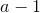and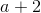. Add and divide by two: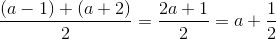### Example Question #4 : Median

Janice's course score in her statistics class depends on her six hourly tests. The mean of her best five scores and the median of her best five scores are both calculated, and she is assigned the better of the two.

Janice's first five tests were 90, 92, 80, 75, and 86. What score, at minimum, does she need to be assured a course score of 85 or better for the term?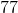Janice is already assured of a course average of at least 85 for the term.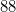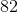Janice is already assured of a course average of at least 85 for the term.

Explanation:

The median of her current five scores is the third-highest, or 86. Even if she does not take the sixth examination, this median will stand, and even if her mean is less, she has already achieved a score of 86 or better.

### Example Question #5 : Median

A data set with nine elements has median 50. A new data set is formed with these nine elements, plus two new elements, 40 and 73.

What is the median of this new data set?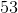It is impossible to tell from the information given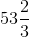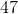Explanation:

The median of the original data set, which has nine elements, is the fifth-highest element; here it is 50. The median of a data set with eleven elements is its sixth-highest element; since one of the elements added is greater than 50 and one is less than 50, 50 becomes the sixth-highest element in the new set, and it remains the median.

### Example Question #1 : Calculating Median

Consider the data set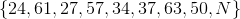whereis a positive integer.

What are the possible values of the median of the set?

38 or 49

37 or 50

Any integer from 38 to 49 inclusive

37, 43.5, or 50

Any integer from 37 to 50 inclusive

Any integer from 37 to 50 inclusive

Explanation:

Arrange the eight known values from least to greatest.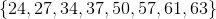If the unknown positive integeris added to the set, then the median of the resulting nine-element set is the fifth-highest.

Case 1:The fifth-highest element is then 37.

Case 2: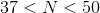The fifth-highest element is then.

Case 3: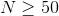The fifth-highest element is then 50.

Therefore, it is possible for the median to be any integer from 37 to 50 inclusive.

### Example Question #1 : Calculating Median

Consider the data set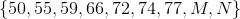For the median to be 70, what must be true aboutand?

One must be equal to 70; the other must be less than or equal to 70.

One must be equal to 70; the other must be greater than or equal to 70.

Both must be equal to 70.

One must be equal to 70; the other must be less than 70.

One must be equal to 70; the other must be greater than 70.

One must be equal to 70; the other must be greater than or equal to 70.

Explanation:

There are nine elements, so the median is the fifth-highest element. For this fifth-highest element to be 70, first of all, 70 must be in the set; since none of the known elements are equal to 70, then one of the two unknowns must be 70.

Assume without loss of generality that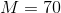. Then four of the elements are already known to be less than 70. Since four elements must be greater than or equal to 70,must be one of them.

Therefore, the correct choice is that one must be equal to 70 and the other must be greater than or equal to 70.

### Example Question #8 : Median

What is the median of the following set: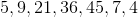Explanation:

Put the numbers in order from least to greatest: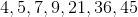The middle number is the median.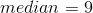### Example Question #71 : Descriptive Statistics

Below is the stem-and-leaf display of a set of test scores.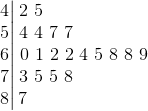What is the median of these scores?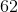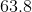Explanation:

The numbers in the "stem" of this display represent tens digits of the test scores, and the numbers in the "leaves" represent the units digits.

This stem-and-leaf display represents twenty scores, so the median is the arithmetic mean of the tenth- and eleventh-highest elements. These elements are 62 and 64, so the median is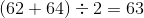.

### Example Question #2081 : Problem Solving Questions

Below is the stem-and-leaf display of a set of test scores.What is the first quartile of these test scores?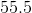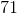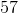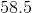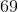Explanation:

The numbers in the "stem" of this display represent tens digits of the test scores, and the numbers in the "leaves" represent the units digits.

This stem-and-leaf display represents twenty scores. The first quartile is the median of the lower half, or the lower ten scores. This is the arithmetic mean of the fifth- and sixth-lowest scores. Both of these scores are the same, however - 57. Therefore, 57 is the first quartile.

← Previous 1 3

### All GMAT Math Resources